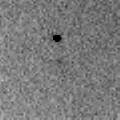# \$B%@%k%+%s%H%sWB@1(B

C/1999 F2 ( Dalcanton )###\$B%W%m%U%#!<%k(B

 \$BH/8+F|(B 1999\$BG/(B 3\$B7n(B20\$BF|(B \$BH/8+8wEY(B 15.5\$BEy(B \$BH/8+ Sloan Digital Sky Survey

###\$B###\$B50F;MWAG(B

```   The following improved orbital elements by Kenji Muraoka, are
from 104 observations 1998 June 18 to 2000 May 5, perturbations
by 9 Planets, Moon and 5 minor planets were taken into account.
The mean residual is +/- 0.72 arc seconds.

Epoch  =  1998 Aug. 15.0  TT       JDT = 2451040.5
T  =  1998 Aug. 23.98570       +/- 0.00309 (m.e.) TT
Peri. =  352.39315                +/- 0.00025
Node  =  210.29598                +/- 0.00028   (2000.0)
Incl. =   56.42109                +/- 0.00018
q  =    4.7187951              +/- 0.0000168 AU
e  =    0.9986054              +/- 0.0000185
1/a  =   +0.0002955              +/- 0.0000039 1/AU
orig. 1/a  =   +0.000054
fut.  1/a  =   +0.000356
```

###\$B@1?^(B###\$B8wEYJQ2=(B

```        m1 = 5.3 + 5 log\$B&\$(B + 10.0 log r
```##### \$B50F;MWAG\$OB<2,7r<#;a\$N7W;;\$K\$h\$k\$b\$N\$G\$9!#(B \$B@1?^\$O(B StellaNavigator Ver.2.0 for Windows (\$B%"%9%H%m%"!<%D(B \$BJTCx(B / \$B%"%9%-!<=PHG6I4)(B) \$B\$G:n@.\$7\$?\$b\$N\$G\$9!#(B \$B8wEY%0%i%U\$O(BComet for Windows\$B\$G:n@.\$7\$?\$b\$N\$G\$9!#(B